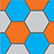Online Program

Thursday, May 30Recent Advances in Matrix and Tensor Factorization Models
Thu, May 30, 4:00 PM - 5:35 PM
Grand Ballroom K

Boosted Sparse and Low-Rank Tensor Regression (305063)

*Kun Chen, University of Connecticut

Keywords: path-following algorithm, regularized estimation, stagewise estimation

We propose a sparse and low-rank tensor regression model to relate a univariate outcome to a feature tensor, in which each unit-rank tensor from the CP decomposition of the coefficient tensor is assumed to be sparse. This structure is both parsimonious and highly interpretable, as it implies that the outcome is related to the features through a few distinct pathways, each of which may only involve subsets of feature dimensions. We take a divide-and-conquer strategy to simplify the task into a set of sparse unit-rank tensor regression problems. To make the computation efficient and scalable, for the unit-rank tensor regression, we propose a stagewise estimation procedure to efficiently trace out its entire solution path. We show that as the step size goes to zero, the stagewise solution paths converge exactly to those of the corresponding regularized regression. The superior performance of our approach is demonstrated on various real-world and synthetic examples.